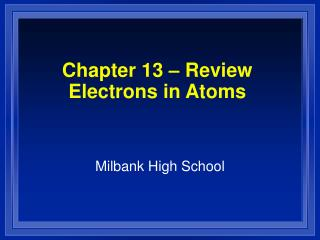DownloadDownload PresentationChapter 13 – Review Electrons in Atoms

# Chapter 13 – Review Electrons in Atoms

Télécharger la présentation## Chapter 13 – Review Electrons in Atoms

- - - - - - - - - - - - - - - - - - - - - - - - - - - E N D - - - - - - - - - - - - - - - - - - - - - - - - - - -
##### Presentation Transcript

1. Chapter 13 – ReviewElectrons in Atoms Milbank High School

2. Chapter 13 - Review • What scientist developed an atomic theory in the early 1800s? • Who discovered the electron? • How does the energy of an electron change when the electron moves closer to the nucleus?

3. Chapter 13 - Review • What scientist developed the quantum mechanical model of the atom? • How many energy sublevels are there in the second principal energy level? • What is the maximum number of d orbitals in a principal energy level?

4. Chapter 13 - Review • What is the maximum number of orbitals in the p sublevel? • What is the maximum number of electrons in the second principal energy level?

5. Chapter 13 - Review • When an electron moves from a lower to a higher energy level, the electron ____ a quantum of energy. • What did Rutherford’s experiment demonstrate? • The quantum mechanical model exactly predicts which characteristic of electrons in an atom?

6. Chapter 13 - Review • What is the probability of finding an electron within the region indicated by the drawn electron cloud? • What types of atomic orbitals are in the third principal energy level?

7. Chapter 13 - Review • Which of the following energy levels has the lowest energy? 3d or 4s • If three electrons are available to fill three empty 2p atomic orbitals, how will the electrons be distributed in the three orbitals?

8. Chapter 13 - Review • What is the next atomic orbital in the series: 1s, 2s, 2p, 3s, 3p, _?_ • How many unpaired electrons are there in a sulfur atom? (atomic number =16)

9. Chapter 13 - Review • What is the number of electrons in the outermost energy level of an oxygen atom? • How many half-filled orbitals are there in a bromine atom? • What is the electron configuration of potassium?

10. Chapter 13 - Review • What statement says that no more than two electrons can occupy an atomic orbital, and that two electrons in the same orbital have opposite spins?

11. Chapter 13 - Review • In order to occupy the same orbital, two electrons must have _____. • According to the Aufbau principle, electrons enter orbitals of _____ energy first.

12. Chapter 13 - Review • Which of the following electron configurations is the most stable? 4d55s1 or 4d45s2 • Stable electron configurations are likely to contain _____.

13. Chapter 13 - Review • Which color of visible light has the shortest wavelength? • What are quanta of light called? • Who predicted that all matter can behave as waves as well as particles?

14. Chapter 13 - Review • The amplitude of a wave is the measure of the ______. • What is the wavelength of an electromagnetic wave that travels at 3 x 108 m/s and has a frequency of 60 Hz? • What is the approximate energy of a photon having a frequency of 4 x 107 Hz? (h = 6.6 x 10-34 J.s)

15. Chapter 13 - Review • What is the approximate frequency of a photon having an energy of 5 x 10-24 J? (h = 6.6 x 10-34 J.s)

16. Chapter 13 - Review • The lowest energy state of an atom is called the ______. • What is the approximate mass of a particle having a wavelength of 1 x 10-7 m, and a speed of 1 m/s? (h = 6.6 x 10-34 J.s)